# Slope Movement

Making platform movement is always a challenge, you have all sorts of nuances, tricks, and wizardry to achieve what you’re looking for. One of those challenges has a name and shape: slopes. But fear nothing, in this post, you’ll understand how to overcome them.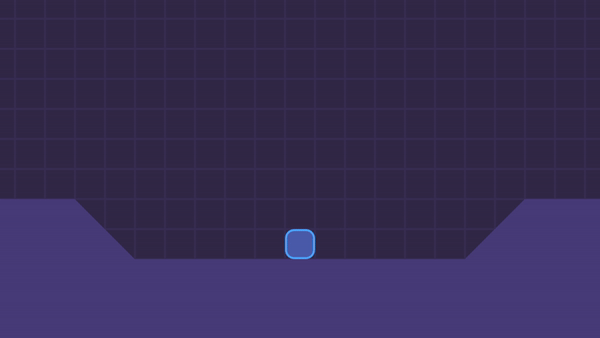## KinematicBody2D is the key

OK, you’re probably using KinematicBody2D already, right? And you are probably using `move_and_slide` as well right? Here is the problem, this is how `move_and_slide` handles slopes: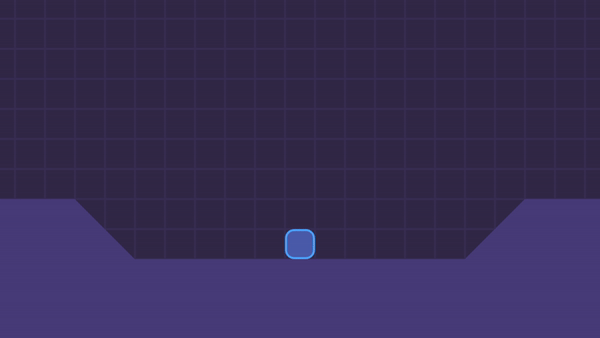Notice how the character seems to fly for a while when it comes from a slope to a flat floor. Also…that’s not all, look how it slides if you stop in the middle of the slope.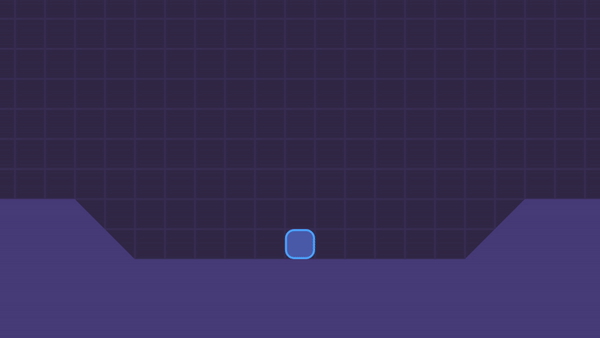You can’t see it just by watching, but as soon as I stopped in the slope on the left, I released the keyboard, all the movement afterward is the remainder of the character trying to climb the slope.

YOU DOUBT IT? Here, try for yourself. This is the code I’m using for this character.

``````extends KinematicBody2D

const FLOOR_NORMAL = Vector2.UP

export(float) var speed = 500.0
export(float) var gravity = 2000.0

var direction = Vector2.ZERO
var velocity = Vector2.ZERO

func _physics_process(delta):
velocity.y += gravity * delta
velocity = move_and_slide(velocity, FLOOR_NORMAL)

func _unhandled_input(event):
if event.is_action("left") or event.is_action("right"):
update_direction()

func update_direction():
direction.x = Input.get_action_strength("right") - Input.get_action_strength("left")
velocity.x = direction.x * speed
if not velocity.x == 0:
sprite.flip_h = velocity.x < 0
``````

### Fixing the Sliding

To fix this sliding is pretty simple. Since the `move_and_slide` method returns the remainder of the movement by using this line:

``````  velocity = move_and_slide(velocity, FLOOR_NORMAL)
``````

We are getting the remainder of the movement and applying as our updated velocity. The remainder of the movement is what remains from a collision. Godot tries to find a way to make your movement possible and the remainder is what Godot couldn’t transform into a valid movement.

So to prevent this remainder to overwrite our horizontal velocity…we simply don’t assign the horizontal remainder. We only use the vertical remainder to allow our character to “climb” the slope:

``````  velocity.y = move_and_slide(velocity, FLOOR_NORMAL).y
``````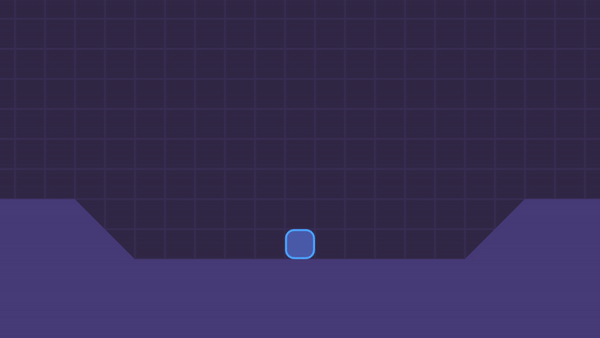You can see that the character still slides a bit if we stop in the middle of the slope. But now is for a different reason. Since we are applying gravity, Godot tries to solve the vertical collision by sliding the character horizontally.

To prevent this, we just need to pass another argument to the `move_and_slide` call. The third argument of the `move_and_slide` method asks you if the character should stop on slopes. So, we can simply say yes or rather `true`.

``````  velocity.y = move_and_slide(velocity, FLOOR_NORMAL, true).y
``````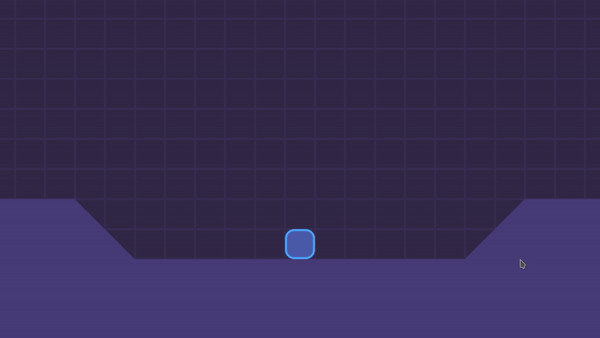### Fixing the hops and floating

Now you can see that the character still performs some “hops” when we stop in the middle of a slope. If we complete the movement, going from a slope to a flat floor, the character will float a bit before it lands on the flat floor. The other way around is true as well. If we come from the flat floor and slide down a slope, the character will float a bit before it lands on the slope.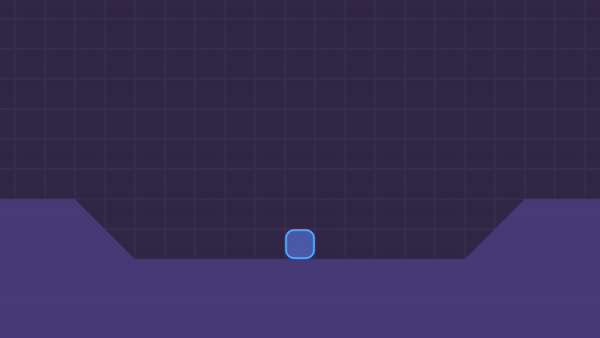This happens because of the inertia of the movement since the movement vector goes upwards and horizontally, the character keeps moving in that direction until enough gravity is applied and it goes down again to the floor.

It looks to me that it would be better if the character…snaps to the floor, right?

It would be awesome if we had such a method, just like `move_and_slide` but that also kept the character snapped to the floor-what? We do?!

Meet `move_and_slide_with_snap`. I think this method was specially created for platform movement because in that context it is an improved version of the `move_and_slide`.

The order of the arguments for this method is a bit different from the `move_and_slide`. We still pass the movement velocity as the first argument, but as the second argument we need to pass…the `snap`!

You can think about the `snap` like a RayCast2D. It is a `Vector2` that needs a direction and a length. As long as this vector touches the floor the character will snap to the ground. This is cool because, if the character falls from a cliff, for instance, it will fall smoothly instead of immediately snaps to the floor below, as long as the `snap` vector doesn’t touch the floor.

So, let’s design the `snap_vector`. For that, we need three things:

• A direction
• The length
• A variable that mixes the two above

You might be thinking:

Why don’t we just use a constant value, like `Vector2(0, 32)`?

Well, it turns out that you may need to change the `snap` vector some times. For instance, if you want the character to jump, you need to turn off the snapping, and one simple way to do that is to turn the `snap` vector into a vector with zero length, i.e. `Vector2.ZERO`.

Continuing… Let’s create those values using the script I’ve put here earlier. At the top, below the `const FLOOR_NORMAL = Vector2.UP` declaration, let’s declare the snap direction and length.

``````const SNAP_DIRECTION = Vector2.DOWN
const SNAP_LENGTH = 32
``````

And below the `var velocity = Vector2.ZERO` line, let’s combine those into the amazing `snap_vector`!

``````var snap_vector = SNAP_DIRECTION * SNAP_LENGTH
``````

Now we can pass this `snap_vector` to the `move_and_slide_with_snap` method and after that, we can pass our other arguments, like the `FLOOR_NORMAL` and whether the character should stop on slopes or not:

``````  velocity.y = move_and_slide_with_snap(velocity, snap_vector, FLOOR_NORMAL, true)
``````

With that our character can walk properly on slopes. Don’t they grow up fast?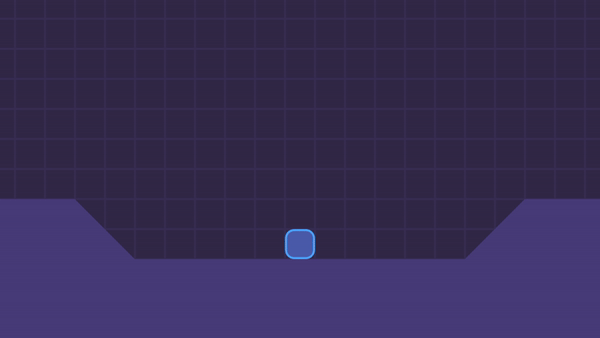Now, you may notice that in the recipes-slope-movement version `1.0.0` I’ve used a `SNAP_THRESHOLD` and passed it as the sixth argument of the `move_and_slide_with_snap` call. I remember there was a reason for that, but it seems like as in Godot Engine `3.2.2` the snapping works without it, so…I think we can ignore(?) at least if your slopes have a maximum angle of 45 degrees like mine.

Just as a reminder, this is how the complete script looks like by the end of this tutorial.

``````extends KinematicBody2D

const FLOOR_NORMAL = Vector2.UP
const SNAP_DIRECTION = Vector2.DOWN
const SNAP_LENGTH = 32

export(float) var speed = 500.0
export(float) var gravity = 2000.0

var direction = Vector2.ZERO
var velocity = Vector2.ZERO
var snap_vector = SNAP_DIRECTION * SNAP_LENGTH

func _physics_process(delta):
velocity.y += gravity * delta
velocity.y = move_and_slide_with_snap(velocity, snap_vector, FLOOR_NORMAL, true).y

func _unhandled_input(event):
if event.is_action("left") or event.is_action("right"):
update_direction()

func update_direction():
direction.x = Input.get_action_strength("right") - Input.get_action_strength("left")
velocity.x = direction.x * speed
if not velocity.x == 0:
sprite.flip_h = velocity.x < 0
``````

That’s it, thank you for reading, keep developing and until the next time!

ps: Let me know if you want a tutorial on how to make the character jump with `move_and_slide_with_snap`

## Files

Slope Movement 12 kB
Version 1.0.0 Jun 25, 2020

## Get Gamedev Experiments

(1 edit)

So If the player needs to jump now, how do you switch off the snapping?

You can read the solution here:

https://pigdev.itch.io/experiments/devlog/164517/jump-with-snap

Thanks. That's exactly what I ended up doing and it worked. Obrigado.

After almost a full day trying to solve this problem using a ton of different combinations of code ... You finally give the right anwer. Thank You very much !!!

Very helpful, but if we get only the Y-axis remainder, if you try to climb too steep slope (like 5-10 degrees), you will be able to climb it, even be pushed in the air.

I’ve just tested with a 4 and a 7 degrees slope and the movement looks fine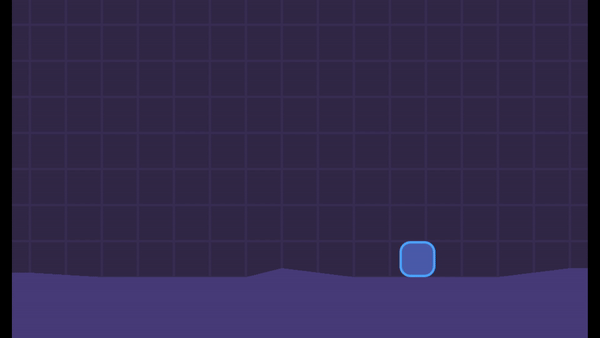Oh sorry, I meant 5-10 degrees difference on already 90 degrees slope.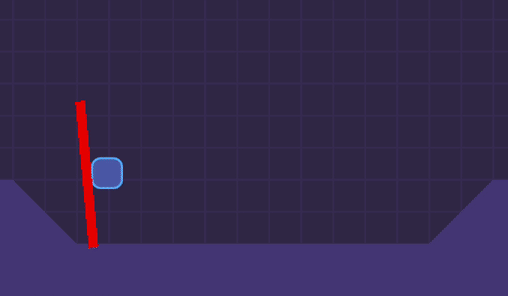Actually, the problem doesn't come from the Y-axis remainder, but the parameter floor_max_angle doesn't seem to work correctly in the function move_and_slide_with_snap and move_and_slide

(+1)

How would I go on about tilting the  sprite depending on the floor?

I have made some attempts in my experimental prototype game. In the end couldn't quite figure out and gave up.

And please do the tut with jump as well.

Hey! Glad to know it helped.

For tilting, I think you could grab the collision normal angle and rotate. But I’m not sure…could you make a request on the community page?

Você é uma inspiração.

Muito obrigado! Fico extremamente feliz sabendo disso <3